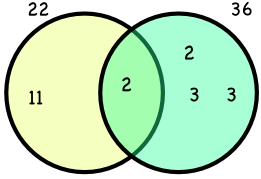Lowest Common Multiple

# Lowest Common Multiple

GCSE(F) GCSE(H)

Multiples can be shared by numbers. The lowest multiple shared by two numbers is called the Lowest Common Multiple, or LCM.

multiples of 9 are: 9, 18, 27, 36, 45, 54, 63, 72, ...

multiples of 12 are: 12, 24, 36, 48, 60, 72, ...

Multiples that appear in both lists are 36 and 72. The Lowest Common Multiple is 36, which is the first multiple that 9 and 12 share.

For larger numbers, it is easier to determine the LCM by using the product of the primes for each number.

Using a Venn Diagram, the union of the two sets represents the lowest common multiple. Higher Students: This can be written as for example) 9 ∪ 12, or 9 union 12.

## Examples

1. What is the LCM of 8 and 10?

By inspection:

Factors of 8: 8, 16, 24, 32, 40, 48, 56, 64, 72, 80

Factors of 10: 10, 20, 30, 40, 50, 60

The lowest common multiple is 40.

2. In a game, one type of alien appears ever 22 seconds. A second type of alien appears every 36 seconds. If they have just appeared together, how long will it be until they appear together again?